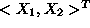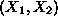Next: The solution space of Up: Graphical solution of 2-var Previous: Graphical solution of 2-var

#### Feasible Regions of Two-Var LP's

The primary idea behind the geometrical representation adopted in the subsequent analysis, is to correspond every vectordenoting the variables of a 2-var LP, to the point with co-ordinatesin a 2-dim (planar) Cartesian system. Under this correspondence, the feasible region of a 2-var LP is depicted by the set of points the coordinates of which satisfy the LP constraints and the sign restrictions. Since all these constraints are expressed by linear inequalities, to geometrically characterize the feasible region, we must first characterize the set of points that constitute the solution space of a linear inequality. Then, the LP feaslible region will result from the intersection of the solution spaces corresponding to each technological constraint and/or sign restriction.

UAL Data
Fri Jun 20 15:03:05 CDT 1997